## Problems, Problems

Saturday 5 May 2007 at 2:22 pm | In Articles | 3 Comments

The Art of Problem Solving (AopS) site encourages, teaches and promotes mathematics competitions from small local ones right up to the International Mathematical Olympiad (IMO). As it says on its front page:

Is math class too easy for you? Looking for a greater challenge?
You’ve come to the right place.

It has an excellent introduction tositeÂ and a forum to discuss problems. The forum has an RSS feed where students pose new problems every day. So if you’re “looking for a greater challenge” subscribe to this feed. Here is a random sample of some of the problems posed recently, which range from very easy to extremely difficult. Click on the problem number to go to the discussion on it.

1. We define addition in a different wayÂ to usual; an addition statement is true only if the letters in the addends is a rearrangement of the letters in the sum. For example,
10 + 6 = 16?
TEN + SIX = TENSIX = SIXTEN, but to be 16 it would need another E.
Find a “true” addition a + b = c + d.

2. Prove that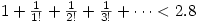. 3. Let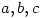be nonzero real numbers. Find all ordered pairs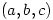such that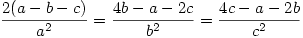.

4.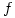is a continuous complex-valued function satisfying:
i)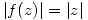ii)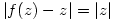Find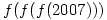5. If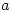and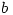are relatively coprime, find all possible values of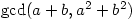.

6. Let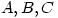be three angles ofÂ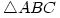. Prove that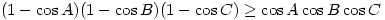.

7. For each functionwhich is defined for all real numbers and satisfies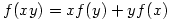and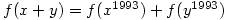determine the value of.

8. Letbe positive reals such that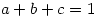. Prove that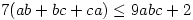.

9. For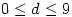, we define the numbers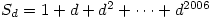. Find the last digit of the number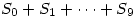.

10. The product of several distinct positive integers is divisible by. Determine the minimum value the sum of such numbers can take.

1. Another similar site is http://www.zadania.info Unfortunately it is in Polish only.

Comment by jan — Monday 23 June 2008 9:20 am #

2. art of problem solving is fantastic. It contains so many challenging math problems. Another good thing is that it is linked to http://www.mathlinks.ro, which have even more hard problems.

Comment by Dave S — Friday 27 June 2008 11:31 am #

3. They are the same sites, just different skins.

Comment by steve — Friday 27 June 2008 12:15 pm #

Sorry, the comment form is closed at this time.